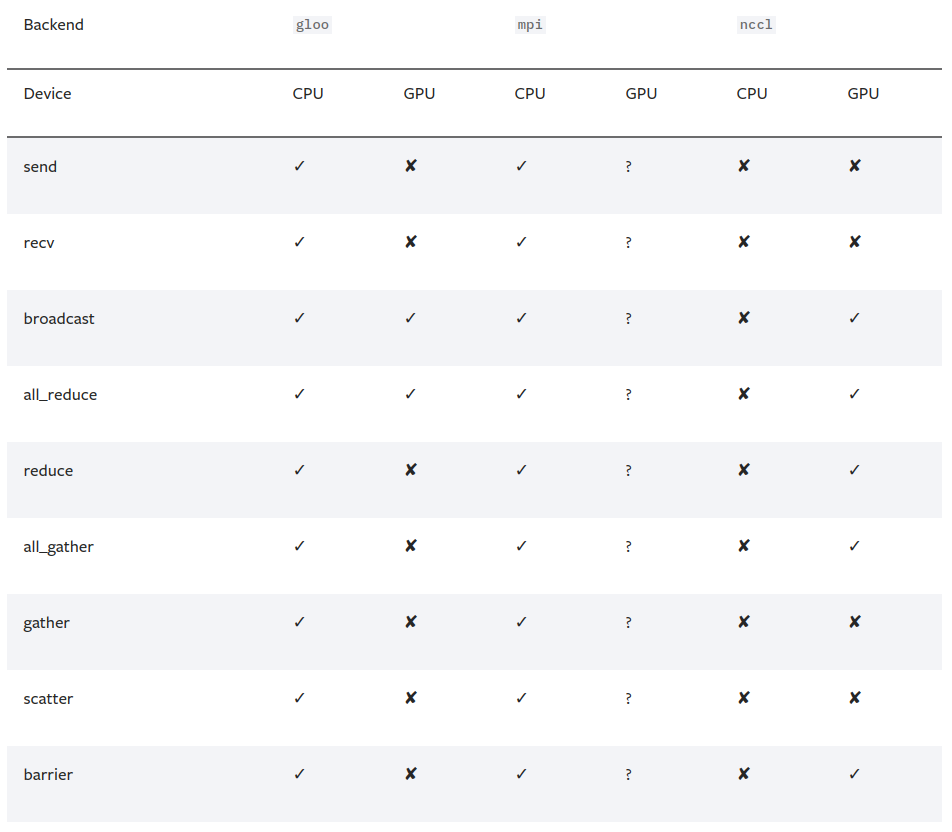## 1. 单机多卡并行训练

### 1.1. torch.nn.DataParallel

os.environ['CUDA_VISIBLE_DEVICES'] = '0,3'

model = nn.DataParallel(model)
model = model.cuda()

inputs = inputs.cuda()
labels = labels.cuda()

model = Model(input_size, output_size)
if torch.cuda.device_count() > 1:
print("Let's use", torch.cuda.device_count(), "GPUs!")
# dim = 0 [30, xxx] -> [10, ...], [10, ...], [10, ...] on 3 GPUs
model = nn.DataParallel(model)

model.to(device)

class DataParallel(Module):
def __init__(self, module, device_ids=None, output_device=None, dim=0):
super(DataParallel, self).__init__()

if not torch.cuda.is_available():
self.module = module
self.device_ids = []
return

if device_ids is None:
device_ids = list(range(torch.cuda.device_count()))
if output_device is None:
output_device = device_ids

### 1.2. 如何平衡DataParallel带来的显存使用不平衡的问题

class BalancedDataParallel(DataParallel):
def __init__(self, gpu0_bsz, *args, **kwargs):
self.gpu0_bsz = gpu0_bsz
super().__init__(*args, **kwargs)
...

my_net = MyNet()
my_net = BalancedDataParallel(gpu0_bsz // acc_grad, my_net, dim=0).cuda()

batch_szie = 8
gpu0_bsz = 2
my_net = MyNet()
my_net = BalancedDataParallel(gpu0_bsz // acc_grad, my_net, dim=0).cuda()

batch_szie = 16
gpu0_bsz = 4
my_net = MyNet()
my_net = BalancedDataParallel(gpu0_bsz // acc_grad, my_net, dim=0).cuda()

### 1.3. torch.nn.parallel.DistributedDataParallel

pytorch的官网建议使用DistributedDataParallel来代替DataParallel, 据说是因为DistributedDataParallelDataParallel运行的更快, 然后显存分屏的更加均衡. 而且DistributedDataParallel功能更加强悍, 例如分布式的模型(一个模型太大, 以至于无法放到一个GPU上运行, 需要分开到多个GPU上面执行). 只有DistributedDataParallel支持分布式的模型像单机模型那样可以进行多机多卡的运算. 当然具体的怎么个情况, 建议看官方文档.

torch.distributed.init_process_group(
backend='nccl',
init_method='tcp://localhost:23456',
rank=0,
world_size=1)

rank是标识主机和从机的, 这里就一个主机, 设置成0就行了.

world_size是标识使用几个主机, 这里就一个主机, 设置成1就行了, 设置多了代码不允许.

torch.distributed.init_process_group(backend="nccl")
model = DistributedDataParallel(model) # device_ids will include all GPU devices by default

python -m torch.distributed.launch main.py

model = model.cuda()
model = nn.parallel.DistributedDataParallel(model)

## 2. 多机多gpu训练

pytorch/examples/imagenet/main.py

Distributed-VGG-F

### 2.1. 初始化

torch.distributed.init_process_group()包含四个常用的参数:

 - backend: 后端, 实际上是多个机器之间交换数据的协议.
 - init_method: 机器之间交换数据, 需要指定一个主节点, 而这个参数就是指定主节点的.
 - world_size: 介绍都是说是进程, 实际就是机器的个数, 例如两台机器一起训练的话, world_size就设置为 2.
 - rank: 区分主节点和从节点的, 主节点为0, 剩余的为了1-(N-1), N为要使用的机器的数量, 也就是world_size.

#### 2.1.1. 初始化backendimport os
# 以下二选一, 第一个是使用gloo后端需要设置的, 第二个是使用nccl需要设置的
os.environ['GLOO_SOCKET_IFNAME'] = 'eth0'
os.environ['NCCL_SOCKET_IFNAME'] = 'eth0'

#### 2.1.2. 初始化init_method

 - 使用TCP初始化

import torch.distributed as dist

dist.init_process_group(backend,
init_method='tcp://10.1.1.20:23456',
rank=rank,
world_size=world_size)

 - 使用共享文件系统初始化

import torch.distributed as dist

dist.init_process_group(backend,
init_method='file:///mnt/nfs/sharedfile',
rank=rank,
world_size=world_size)

#### 2.1.4. 初始化中一些需要注意的地方

python distributed.py -bk nccl -im tcp://10.10.10.1:12345 -rn 0 -ws 2

python distributed.py -bk nccl -im tcp://10.10.10.1:12345 -rn 1 -ws 2

print("Initialize Dataloaders...")
# Define the transform for the data. Notice, we must resize to 224x224 with this dataset and model.
transform = transforms.Compose(
[transforms.Resize(224),
transforms.ToTensor(),
transforms.Normalize((0.5, 0.5, 0.5),
(0.5, 0.5, 0.5))
])

trainset = datasets.STL10(root='./data',
split='train',
transform=transform)
valset = datasets.STL10(root='./data',
split='test',
transform=transform)

# Create DistributedSampler to handle distributing the dataset across nodes when training
# This can only be called after torch.distributed.init_process_group is called
# 这一句就是和平时使用有点不一样的地方
train_sampler = torch.utils.data.distributed.DistributedSampler(trainset)

# Create the Dataloaders to feed data to the training and validation steps
trainset,
batch_size=batch_size,
shuffle=(train_sampler is None),
num_workers=workers,
pin_memory=False,
sampler=train_sampler)
valset,
batch_size=batch_size,
shuffle=False,
num_workers=workers,
pin_memory=False)

### 2.3. 模型的处理

model = model.cuda()
model = nn.parallel.DistributedDataParallel(model)

### 2.4. 模型的保存与加载

pytorch官方教程的一段代码:

def demo_checkpoint(rank, world_size):
setup(rank, world_size)

# setup devices for this process, rank 1 uses GPUs [0, 1, 2, 3] and
# rank 2 uses GPUs [4, 5, 6, 7].
n = torch.cuda.device_count() // world_size
device_ids = list(range(rank * n, (rank + 1) * n))

model = ToyModel().to(device_ids)
# output_device defaults to device_ids
ddp_model = DDP(model, device_ids=device_ids)

loss_fn = nn.MSELoss()
optimizer = optim.SGD(ddp_model.parameters(), lr=0.001)

CHECKPOINT_PATH = tempfile.gettempdir() + "/model.checkpoint"
if rank == 0:
# All processes should see same parameters as they all start from same
# random parameters and gradients are synchronized in backward passes.
# Therefore, saving it in one process is sufficient.
torch.save(ddp_model.state_dict(), CHECKPOINT_PATH)

# Use a barrier() to make sure that process 1 loads the model after process
# 0 saves it.
dist.barrier()
# configure map_location properly
rank0_devices = [x - rank * len(device_ids) for x in device_ids]
device_pairs = zip(rank0_devices, device_ids)
map_location = {'cuda:%d' % x: 'cuda:%d' % y for x, y in device_pairs}

outputs = ddp_model(torch.randn(20, 10))
labels = torch.randn(20, 5).to(device_ids)
loss_fn = nn.MSELoss()
loss_fn(outputs, labels).backward()
optimizer.step()

# Use a barrier() to make sure that all processes have finished reading the
# checkpoint
dist.barrier()

if rank == 0:
os.remove(CHECKPOINT_PATH)

cleanup()

## 3. 参考文档

 - Distributed-VGG-F

Last modification：February 13th, 2020 at 03:37 pm